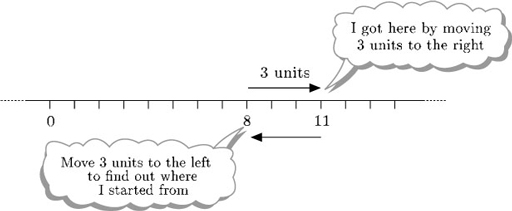Now that you have rules for addition and subtraction of negative numbers, think about multiplication and division.

## Example 27

Describe each of the following in terms of the number line and the value of Thomas's piggy bank:

• (a) the mul
Author(s): The Open University

Now what about subtraction? You can think of subtraction as undoing addition: adding 3 to 8 gets you 11, and so subtracting 3 from the answer, 11, gets you back to 8. Therefore, in terms of the number line, subtracting 3 from 11 means starting at 11 and moving 3 units to the left.Author(s): The Open University

## Activity 52

Evaluate each of the following.

• [Image_Link]https://www.open.edu/openlearn/ocw/pluginfile.php/11742
Author(s):
The Open University

How about other fractions? What is 6 ÷? This means how many [Image_Link]https://www.open.edu/openlearn/ocw/pluginfile.php/117429/mod_ou
Author(s):
The Open University

## Activity 30

Carry out the following calculations, without your calculator.

• (a) 3 × (60 + 70).

• (b) (3 × 60) + 70.

• (c) (70 − 60) ÷ 5.

• Author(s): The Open University

## Activity 28

Look at the rules in the boxes above.

Write in your own words the rules for multiplying and dividing, adding and subtracting two numbers, giving an example of each.

Author(s): The Open University

In order to compare quantities, it is best to express them in the same units.

## Example 10

Three children have just measured their own heights in metric units. Isaac says ‘My height is 1098’, Jasmine says ‘My height is 112’ and Kim says ‘Mine is 1.1’. What units were
Author(s): The Open University

## Activity 15

Suggest appropriate units for each of the following:

• (a) the age of the kitten when it is weaned;

• (b) the distance between one train station and the
Author(s): The Open University

## Activity 9

The diagram below shows an oatmeal cake marked into 12 equal portions. I want to give my sister a third of the cake. Where could I cut the cake, and what would be left over?

Author(s): The Open University

In the example below of a straightforward subtraction, in every column the digit at the top of the column is bigger than the digit at the bottom. Click on each step in turn to see how to carry out the calculation.

Active content not displayed. This content requires JavaScript to be enabled.